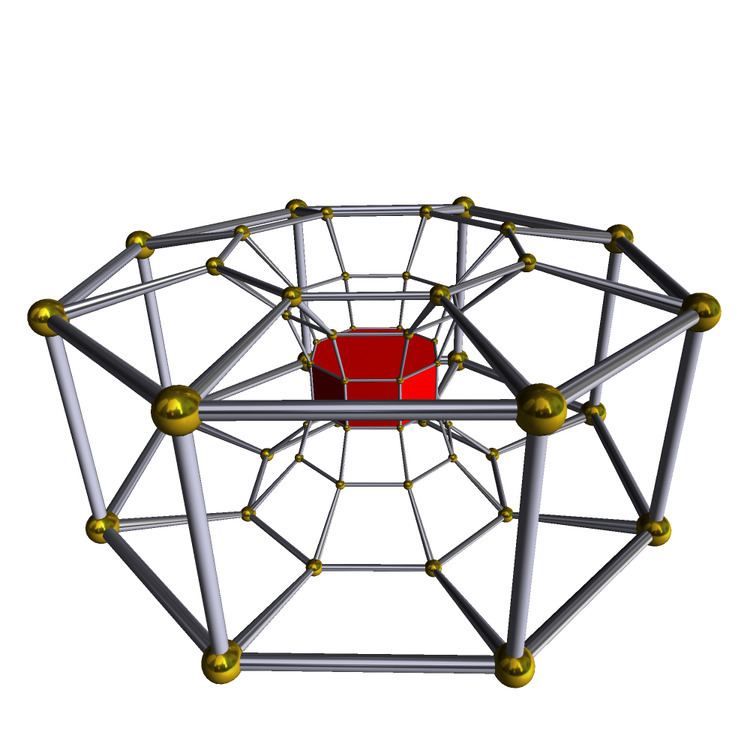# 8 8 duoprism

Updated on
Covid-19In geometry of 4 dimensions, a 8-8 duoprism is a polygonal duoprism, a 4-polytope resulting from the Cartesian product of two octagons.

## Contents

It has 64 vertices, 128 edges, 80 faces (64 squares, and 16 octagons), in 16 octagonal prism cells. It has Coxeter diagram , and symmetry [[8,2,8]], order 512.

## Images

The uniform 8-8 duoprism can be constructed from × or × symmetry, order 256 or 64, with extended symmetry doubling these with a 2-fold rotation that maps the two orientations of prisms together. These can be expressed by 4 permutations of uniform coloring of the octahedral prism cells.

Seen in a skew 2D orthogonal projection, it has the same vertex positions as the hexicated 7-simplex, except for a center vertex. The projected rhombi and squares are also shown in the Ammann–Beenker tiling.

The regular complex polytope 8{4}2, , in C 2 has a real representation as a 8-8 duoprism in 4-dimensional space. 8{4}2 has 64 vertices, and 16 8-edges. Its symmetry is 82, order 128.

It also has a lower symmetry construction, , or 8{}×8{}, with symmetry 88, order 64. This is the symmetry if the red and blue 8-edges are considered distinct.

## 8-8 duopyramid

The dual of a 8-8 duoprism is called a 8-8 duopyramid. It has 64 tetragonal disphenoid cells, 128 triangular faces, 80 edges, and 16 vertices.

The regular complex polygon 2{4}8 has 16 vertices in C 2 with a real represention in R 4 matching the same vertex arrangement of the 8-8 duopyramid. It has 64 2-edges corresponding to the connecting edges of the 8-8 duopyramid, while the 16 edges connecting the two octagons are not included.

The vertices and edges makes a complete bipartite graph with each vertex from one octagon is connected to every vertex on the other.

8-8 duoprism Wikipedia

Topics